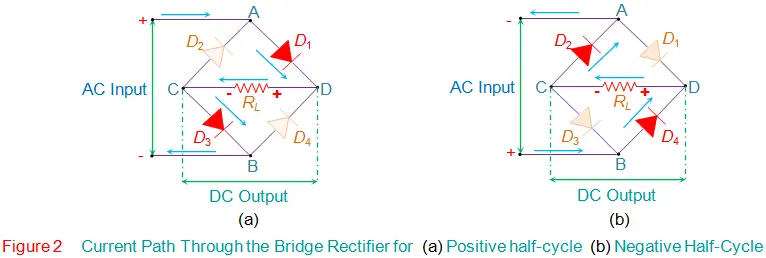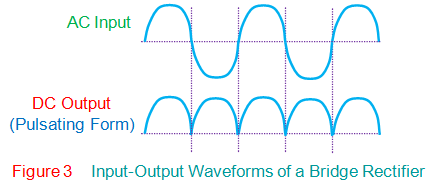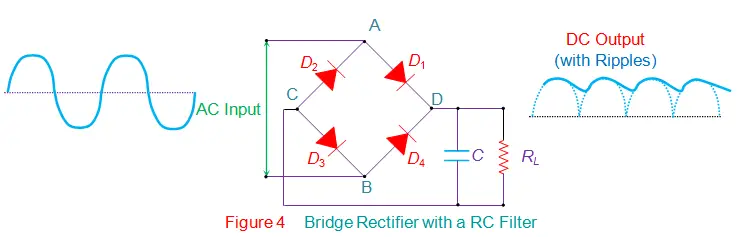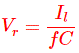# Bridge Rectifiers: What is it? (Circuit Diagram & Working Principle)

## What is a Bridge Rectifier?

Bridge Rectifiers are circuits that convert alternating current (AC) into direct current (DC) using diodes arranged in the bridge circuit configuration. Bridge rectifiers typically comprise of four or more diodes. The output wave generated is of the same polarity irrespective of the polarity at the input.

Bridge rectifiers are in the same class of electronics as half-wave rectifiers and full-wave rectifiers. Figure 1 shows such a bridge rectifier composed of four diodes D1, D2, D3, and D4 in which the input is supplied across two terminals A and B in the figure while the output is collected across the load resistor RL connected between the terminals C and D.

Now consider the case wherein the positive pulse appears at the AC input i.e. the terminal A is positive while the terminal B is negative. This causes the diodes D1 and D3 to get forward biased and at the same time, the diodes D2 and D4 will be reverse biased.

As a result, the current flows along the short-circuited path created by the diodes D1 and D3 (considering the diodes to be ideal), as shown by Figure 2a. Thus the voltage developed across the load resistor RL will be positive towards the end connected to terminal D and negative at the end connected to the terminal C.Next, if the negative pulse appears at the AC input, then terminals A and B are negative and positive respectively. This forward biases the diodes D2 and D4, while reverse biasing D1 and D3 which causes the current to flow in the direction shown by Figure 2b.

At this instant, one has to note that the polarity of the voltage developed across RL is identical to that produced when the incoming AC pulse was positive in nature. This means that for both positive and negative pulse, the output of the bridge rectifier will be identical in polarity as shown by the waveforms in Figure 3.However, it is to be noted that the bridge rectifier’s DC will be pulsating in nature. In order to obtain a pure form of DC, one has to use a capacitor in conjunction with the bridge circuit (Figure 4).In this design, the positive pulse at the input causes the capacitor to charge through the diodes D1 and D3. However as the negative pulse arrives at the input, the charging action of the capacitor ceases and it starts to discharge via RL.

This results in the generation of DC output which will have ripples in it as shown in the figure. This ripple factor is defined as the ratio of the AC component to the DC component in the output voltage. In addition, the mathematical expression for the ripple voltage is given by the equationWhere,
Vr represents the ripple voltage.
f represents the frequency of the ripple which will be twice the input frequency.
C is the Capacitance.

Further, the bridge rectifiers are primarily of two types, viz., Single-Phase Rectifiers and Three-Phase Rectifiers. In addition, each of these can be either Uncontrolled or Half-Controlled, or Full-Controlled.

Bridge rectifiers for a particular application are selected by considering the load current requirements. These bridge rectifiers are quite advantageous as they can be constructed with or without a transformer and are suitable for high voltage applications.

However, here two diodes will be conducting for every half-cycle and thus the voltage drop across the diodes will be higher. Lastly one has to note that apart from converting AC to DC, bridge rectifiers are also used to detect the amplitude of modulated radio signals and to supply polarized voltage for welding applications.

Want To Learn Faster? 🎓
Get electrical articles delivered to your inbox every week.
No credit card required—it’s 100% free.